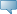# Tennessee Technological University

## Catalog EntriesSelect the Course Number to get further detail on the course. Select the desired Schedule Type to find available classes for the course.

 MATH 1530 - Introductory Statistics Descriptive statistics including measures of central location and variation, frequency distributions, histograms, and frequency polygons. Probability relating to elementary sample spaces, events, conditional probability, discrete and continuous type random variables, mathematical expectation, and the normal probability. Inferential statistics relating to the confidence intervals and hypothesis tests related to the mean and proportion. 3.000 Credit hours 3.000 Lecture hours Levels: Undergraduate Schedule Types: Lecture Mathematics Department Course Attributes: General Education-Math, Geometry Advanced Math